# All About Feed-Forward network with python code

## Overview

The Feed-Forward network is the first half of the neural network in which we predict our first output. In order to learn a feed-forward network, we have to learn the scaling of data for data manipulation, the Activation function for extracting important information, and the structure of the feed-forward network.

## Topics Covered

1. Scaling In Machine Learning
2. Sigmoid Activation Function
3. Softmax Activation Function
4. Structure of Feed-Forward Network
5. Matrix Representation of Weights
6. Code of the Feed-Forward Network

## Scaling In Machine Learning

Let us say there are two values in a row or a column

1. 3000 m
2. 3 km

Now both the values have the same meaning, but our machine learning model does not know that therefore 3000 m will be a higher value and 3 km would be a smaller value in comparison for our model and we don’t want that. In order to standardize the independent features, we are going to scale our data which will convert the values within the range of 0 to 1.

Most Used Scaling Techniques :

1. Min-Max Normalization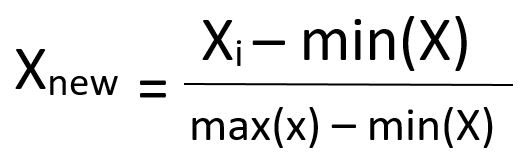1. Standardization## Sigmoid Activation Function

Activation functions add non-linearity to the model which helps in decision-making through layers.

Sigmoid activation function compresses the output in the range of 0 to 1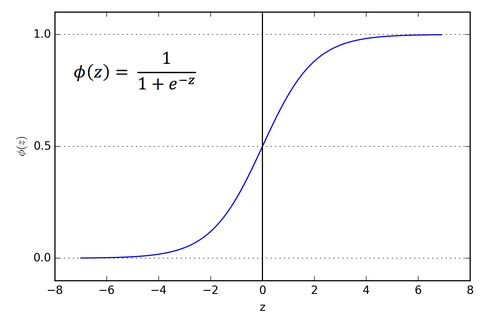## Softmax Activation Function

Softmax Activation Function is basically used in the output layer to make the final decision about the relevancy of data.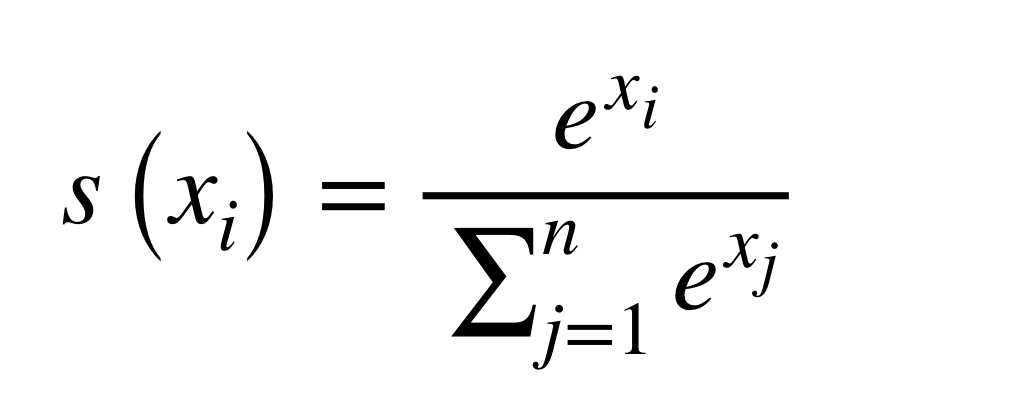## Structure of Feed-Forward Network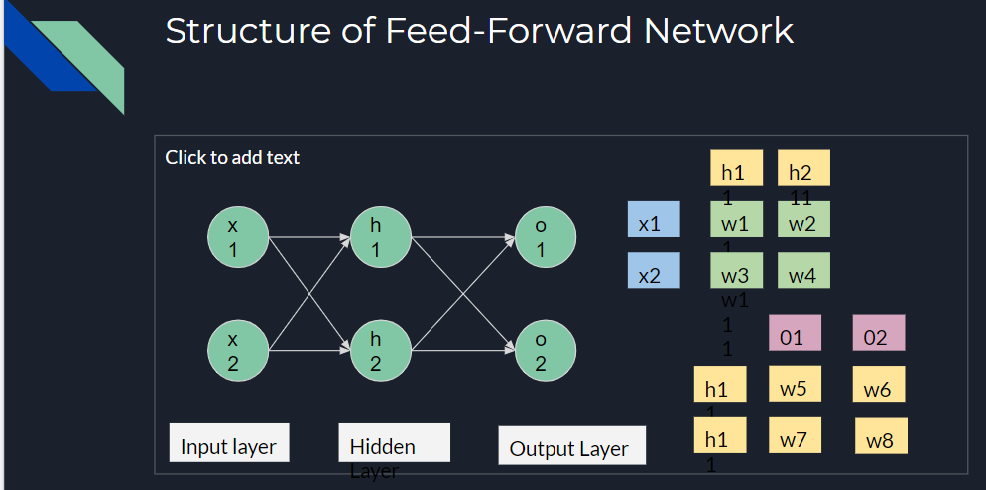From the above structure, we can conclude that the feed-forward network has an input layer, the hidden layer(s), and an output layer. A structure of the Feed-forward network without a hidden layer is known to be a perceptron.

Also, there can be ‘n’ numbers of hidden layers present in a network.

You can notice the matrix representation of how the weights have been allotted in between the layers. Below is their representation -:

(x1 , h1) = w1                (h1 , o1)  = w5

(x1 , h2) = w2                (h1 , o2) = w6

(x2 , h1) = w3                 (h2 , o1) = w7

(x1 , h2) = w4                 (h2 , o2) = w8

## Matrix Representation of Weights in a Neural Network

Let us suppose the value of the input layer is 6 and 5 respectively, therefore, the matrix representation of the information would look like

-: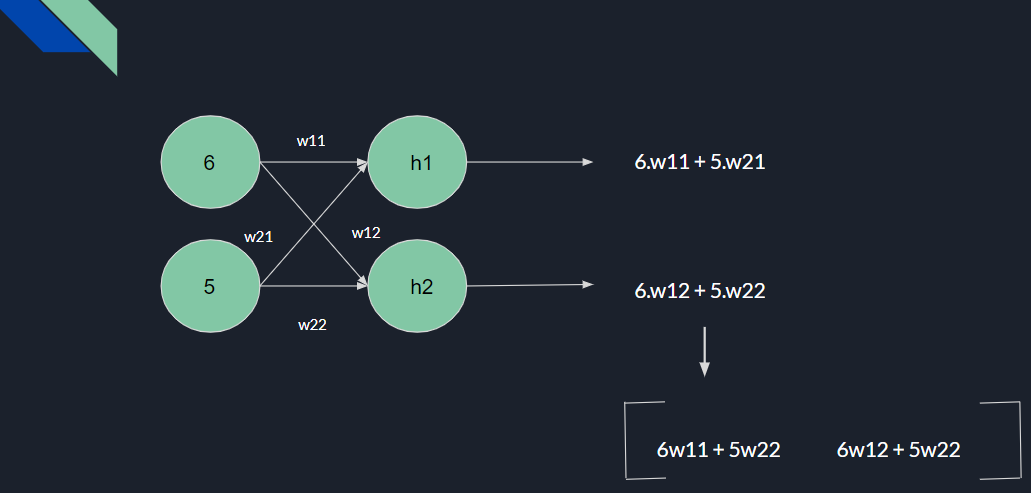In this way, representation becomes so easy that no matter how many rows our input layer has, it will be represented in an organized way. Also, the representation is the same in the case of the hidden layer and output layer too.

After each layer, we have to initialize an activation function which we have discussed above.

So now we shall see the matrix representation at the output layer -: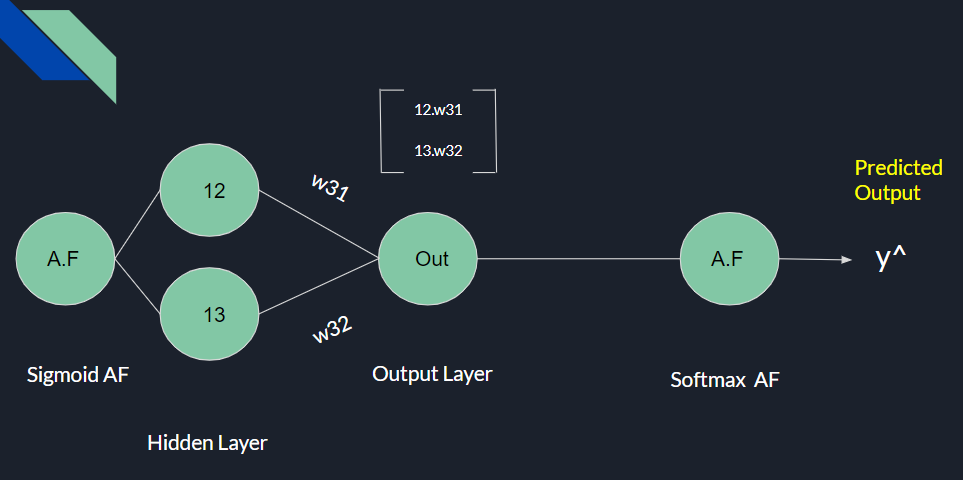This is how we predict the output initially, Although our accuracy on our first prediction would be poor, so we need to update the weights and biases to improve our accuracy with the help of the Backpropagation Algorithm in which we compute loss and then update our weights with a certain learning rate. But for the feed-forward network, this is it.

## Feed-Forward Network Python Code Implementation

``````import numpy as np
import matplotlib.pyplot as plt
import pandas as pd

dataset = dataset.values
dataset.shape``````
`Out: (42000, 785)`

## Scaling

``````# Min-Max Scaler

X = (X - X.min()) / (X.max() - X.min())``````

## Initializing Input layer, Activation Function, and Feed-Forward network

``````class NeuralNetwork:

def __init__(self, X, y):
self.X = (X - X.min()) / (X.max() - X.min())
self.y = y
self.H1_size = 256
self.H2_size = 64
self.OUTPUT_SIZE = len(np.unique(y))
self.INPUT_SIZE = X.shape
self.losses = []

# Initialize weights
self.W1 = np.random.randn(self.INPUT_SIZE, self.H1_size)
self.W2 = np.random.randn(self.H1_size, self.H2_size)
self.W3 = np.random.randn(self.H2_size, self.OUTPUT_SIZE)

# Initialize biases
self.b1 = np.random.random((1, self.H1_size))
self.b2 = np.random.random((1, self.H2_size))
self.b3 = np.random.random((1, self.OUTPUT_SIZE))

def sigmoid(self, z):
return 1 / (1 + np.exp(-z))

def sigmoid_prime(self, z):
s = self.sigmoid(z)
return s * (1 - s)

def softmax(self, z):
return np.exp(z) / np.sum(np.exp(z), axis=1, keepdims=True)

def forward(self, x):
Z1   = x.dot(self.W1) + self.b1 # (N,256) = (N,784)(784,256)(1,256)
A1   = self.sigmoid(Z1)
Z2   = A1.dot(self.W2) + self.b2
A2   = self.sigmoid(Z2)
Z3   = A2.dot(self.W3) + self.b3
yhat = self.softmax(Z3)

self.activations = [A1, A2, yhat]

return yhat``````

## Ending-Notes